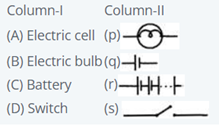Physics
Easy

Question

# DIRECTIONS: Match Column-I with Column-II and select the correct answer using the codes given below the columns.## A→ (p), B→ (q), C→ (r), D→ (s)A→ (p), B→ (q), C→ (s), D→ (r)A→ (q), B→ (p), C→ (r), D→ (s)A→ (q), B → (p), C→ (s), D→ (r)Hint:

## The correct answer is: A→ (q), B→ (p), C→ (r), D→ (s)

### A→ (q), B→ (p), C→ (r), D→ (s)matching with their respective symbols.p represents bulb, q represents cell, r is group of cells which is battery, s represents switch.#### With Turito Foundation.#### Get an Expert Advice From Turito.